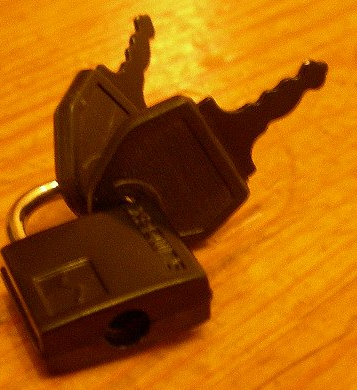# 24x Speedup—  1 year, 10 months ago
Here is how I measure the speed of my program: I run the game for 1 000 000 randomly picked tetrominoes and measure how much time it takes. In the js prototype it takes 40 seconds.

Here is what I have done in May.

1. Implemented the matching algorithm in C. Running time was 44 seconds, slightly worse than the js version. Compiling with /O2 gave a 2x speedup, bringing it down to around 23 seconds.

2. Made it possible to test a program by loading a pre-generated sequence of tetrominoes and turning on deterministic mode (it was randomized in several ways). Now the game runs identically between launches. After making a change I can make sure that the game runs the program the same way it ran it before by just comparing two files that store results of the run.

3. Improved efficiency of the matching algorithm. Instead of trying to match the pattern to every region of the field, now the game only tries to match it to the regions on the surface. Here is what I mean by that. Let's assume that a tile of the pattern is full and all tiles above it are guaranteed to be empty. Then if that pattern ever matches a region of the field, this tile will be matched to the highest full tile in one of the columns of the field.Therefore, you can calculate the surface of the field (one tile in each column, such that all tiles above that tile are empty) and only match the surface of the pattern to the surface of the field. Yellow tiles on the following picture are the surface of the field.I take the tiles under the intended position of the tetromino as the surface of a pattern, because the matching algorithm guarantees that all tiles above the tetromino are empty (otherwise the tetromino won't always land where the player expects it to land).All columns that don't contain the tetromino aren't guaranteed to be empty, regardless of the structure of the pattern. Here is a simple example.Because of that, it's impossible to apply this optimization to floorless patterns, because they don't have any surface.The surface function sets the surface to the tiles right below the tetromino, but it also recognizes that it is outside of the pattern, and the matching algorithm uses the old way (trying to match everywhere). Nevertheless, this improvement yielded more that 2x speedup, bringing the runtime down to about 10 seconds. Floorless patterns get hit two times less frequently than floored patterns and take about twice longer (combined) to get matched. Therefore, if you rewrite the program so that it doesn't use floorless patterns, I expect it to give another 2x speedup (although, the program will become worse).

4. Changed the format of data. The field was previously a 2D array of integers, where 0 means that the tile is empty, and numbers 1 - 8 mean different colors. Now it is a 1D array of uint32, where each number is a row, and each bit of that number is a tile in that row. 0 means empty, 1 means full, no colors.With this format, matching two rows means taking a bitwise "xor" of them. If the result is 0, then the rows are the same. There is a little caveat with patterns, though. Patterns contain tiles that are full, tiles that are empty and tiles that can be either (in the js prototype they are marked by question marks). The pattern will match a region regardless of whether the corresponding tiles of the field are empty.To make the new pattern representation reflect that fact, I keep two sets of tiles for each pattern: mask tiles and data tiles. Mask tiles are zero if the original tile is a question mark and one otherwise. Data tiles are one if the original tile is full and zero otherwise. For the following patternthe data tiles will beand the mask tiles will beMatching a row of the field and a row of the pattern, then, consists of two steps. First, taking a bitwise "and" of the field row and the mask row. Second, taking a bitwise "xor" of the result of the first step and the data row. If the result of the second step is zero, then the rows match. This brought the runtime down to exactly 2 seconds (before this step I used a regular stopwatch to measure the time, so the result wasn't very precise).

5. Measured how much time every part of the algorithm takes. As expected, out of these 2 seconds looking for matches takes 1.8 seconds, 1.7 of which is spent in the function that matches a pattern to a specific region of the field.

6. Converted the matching function to SIMD. Now that the function operates on rows of the field and rows of the pattern, I can match 4 rows at once. This brought the runtime down to 1670 ms, which is almost 24x speedup compared to the prototype.

Here is what I haven't done.

1. I haven't tried to make the computation parallel. Since most of the time you need to try several patterns before you find one that matches anything, you can just as well try to match several patterns in parallel. However, I don't really know much about parallel computations, so I will explore this direction later.

2. I haven't optimized the function that calculates the surface of the field. It takes 120 ms per million tetrominoes, so it might help a little bit.

70 hours, 1500 lines. The core game mechanic is finished, the plan for June is to start implementing the UI.—  1 year, 10 months ago
Exciting update! Looks like you're getting things done. :)—  1 year, 10 months ago
What a fun read. Optimizations like this are fun to read about, especially when you have hard numbers about how it improved.

Cool stuff.—  1 year, 10 months ago
Thanks, I'm glad you liked it.